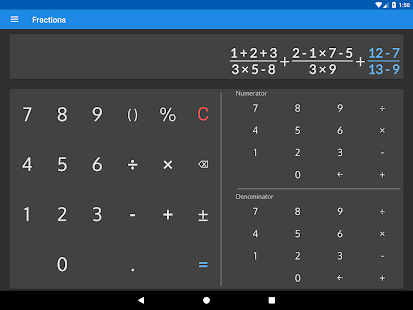Best and free offline fraction calculator, supporting also decimals, percentages, and parentheses that shows a detailed solution for all calculations.

At school or home need to solve simple or complex math fraction problems, convert fractions into decimals, or decimals into fractions? – this fraction calculator will do it and will show how it was done

Perfect for students, parents, teachers, and woodworkers to solve or verify fraction everyday problems.

Features of this fraction calculator free app

Supports combining decimal and fractional numbers. Automatically reduces fraction result to its simplest form.
Converts fractions into decimals and decimals into fractions. Calculated result is displayed using fractions. Equivalent value in decimals is also available.
Shows a detailed solution, step by step for all fraction problems and calculations.
History of previous calculations is available.
Besides basic math operations such as add, subtract, multiply and divide; fraction calculator allows calculating percentages for fractions.

Works with big numbers. No limits

We have made this fraction calculator app available in multiple colors for you!

WHAT’S NEW

• Improved solution
• Bug fixes

Screenshots

•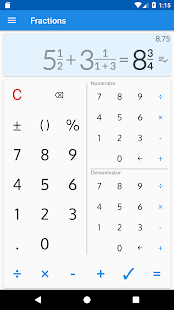•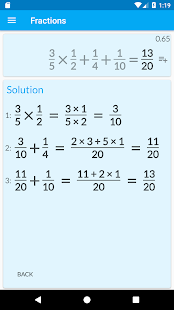•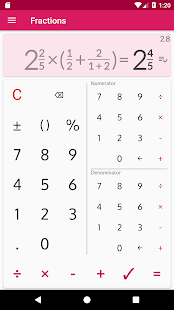•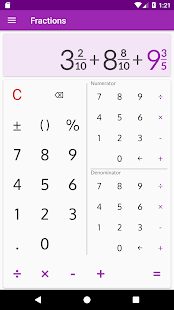•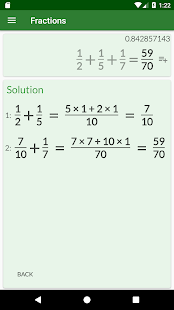•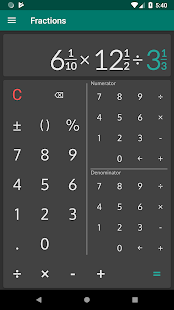•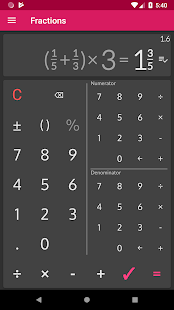•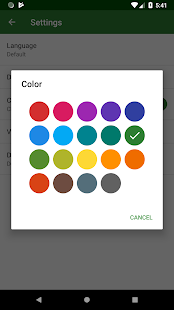•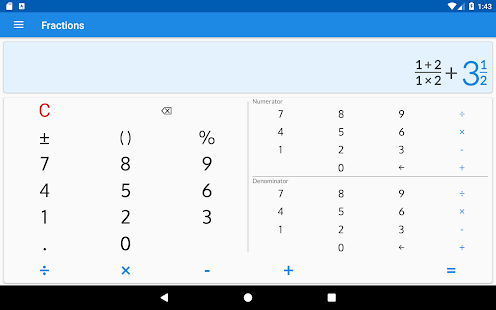•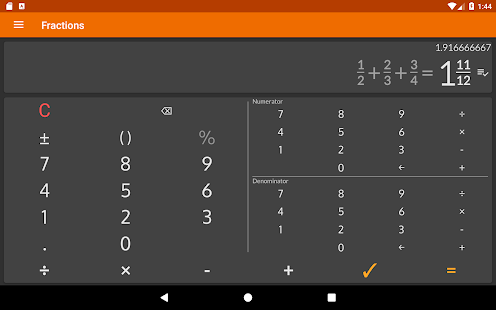•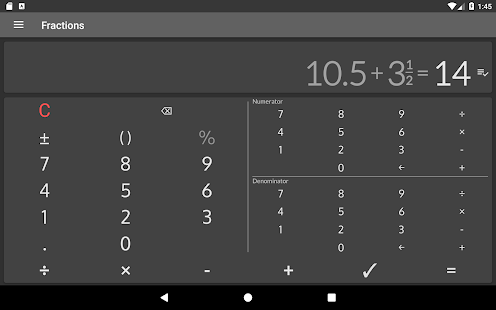•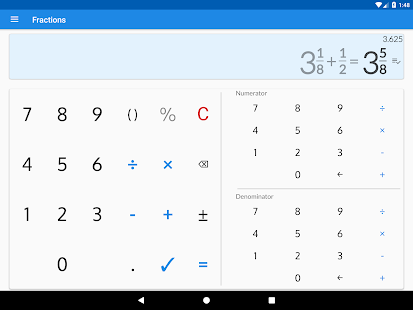•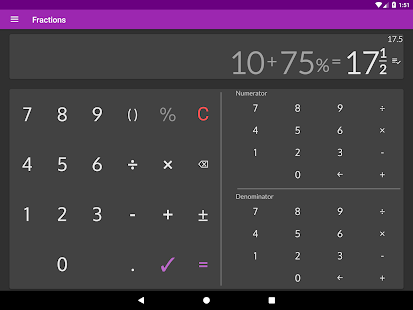•